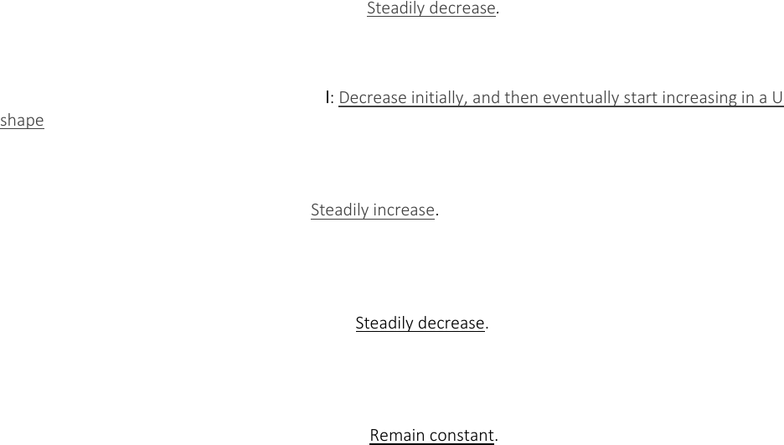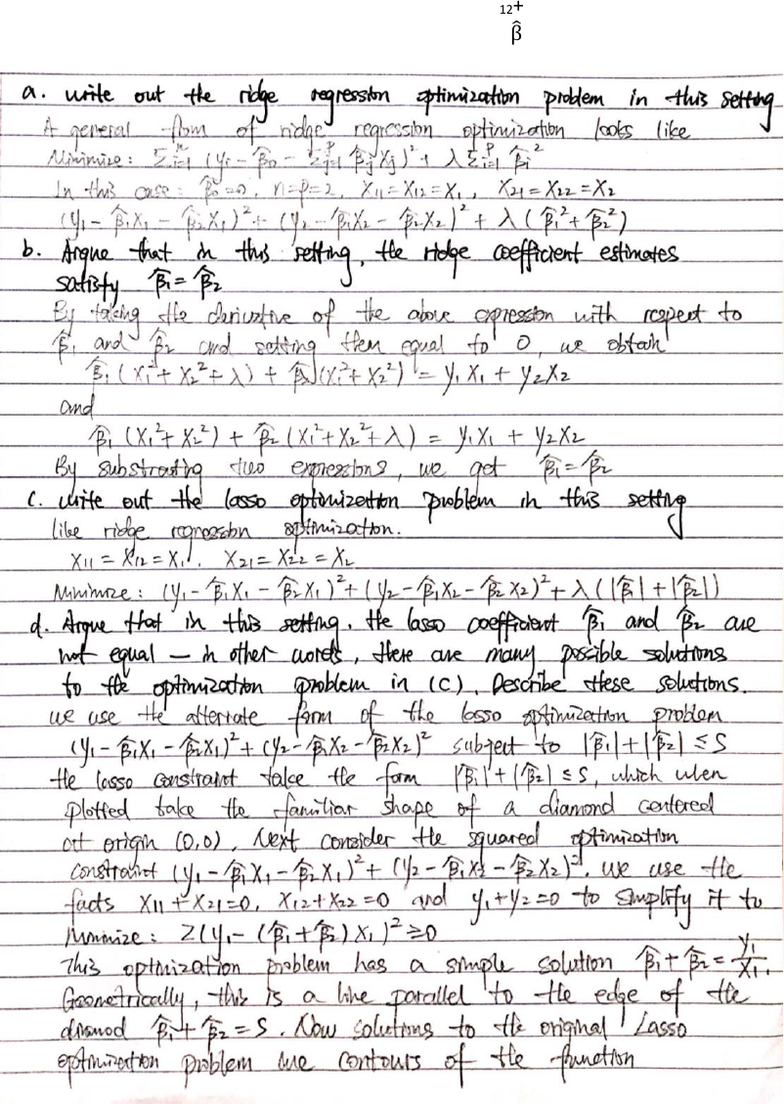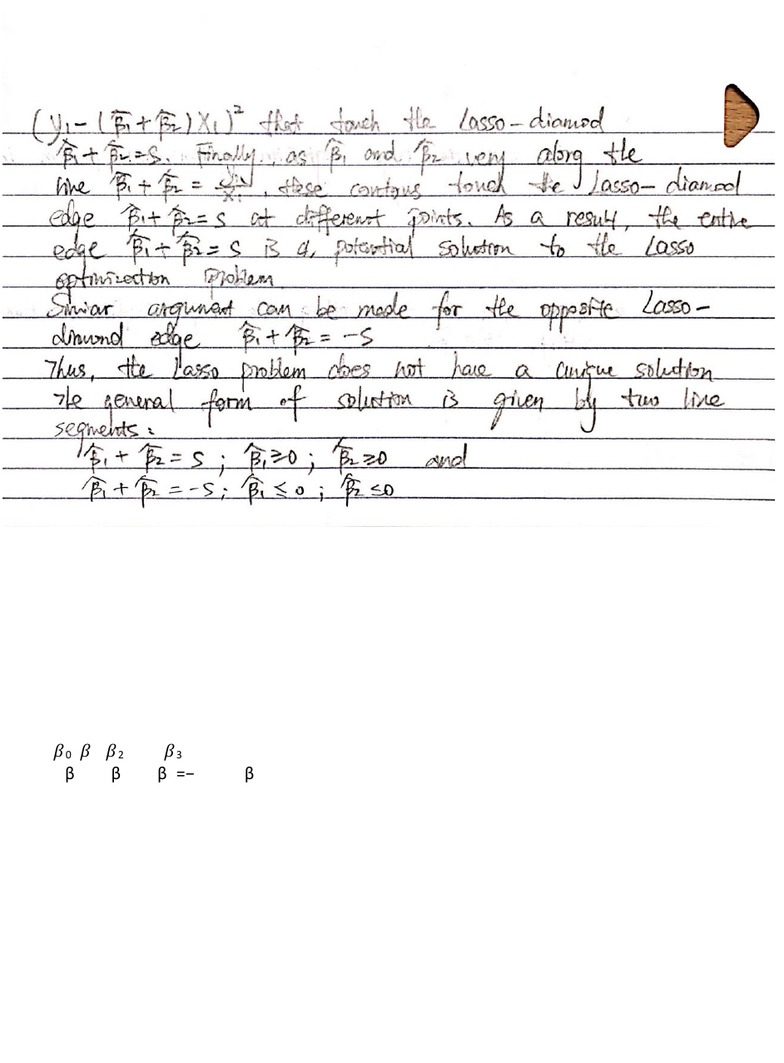# ART111 Lecture Notes - Lecture 2: Bayes Error Rate, Overfitting, Tikhonov Regularization

45 views11 pagesHomework 3 Due data 10/25/2018
Question 1: Suppose we estimate the regression coefficients in a linear regression model by minimizing
(𝑦
𝑛
𝑖=1 I β0 - 𝛽
𝑝
𝑗=1 j xij)
subject to |𝛽
𝑝
𝑗=1 j| ≤ s for a particular value of s.
(a) As we increase s from 0, the training RSS will: Steadily decrease. As we increase s from 0, all β's
increase from 0 to their least square estimate values. Training error for 0 βs is the maximum and it
(b) As we increase s from 0, the test RSS will: Decrease initially, and then eventually start increasing in a U
shape. When s=0, all βs are 0, the model is extremely simple and has a high test-RSS. As we increase s, βs
assume non-zero values and model starts fitting well on test data and so test RSS decreases. Eventually,
as βs approach their full blown OLS values, they start overfitting to the training data, increasing test-RSS.
(c) As we increase s from 0, variance will: Steadily increase. When s=0, the model effectively predicts a
constant and has almost no variance. As we increase s, the models include more βs and their values start
increasing. At this point, the values of βs become highly dependent on training data, thus increasing the
variance.
(d) As we increase s from 0, (squared) bias will: Steadily decrease. When s=0, the model effectively
predicts a constant and hence the prediction is far from actual value. Thus, bias is high. As s increases,
more βs become non-zero and thus the model continues to fit training data better. And thus, bias
decreases.
(e) As we increase s from 0, Bayes error rate will: Remain constant. By definition, irreducible error is
model independent and hence irrespective of the choice of s, remains constant.
Unlock document

This preview shows pages 1-3 of the document.
Unlock all 11 pages and 3 million more documents.Question 2: It is well-known that ridge regression tends to give similar coefficient values to correlated
variables, whereas the lasso may give quite different coefficient values to correlated variables. We will
now explore this property in a very simple setting. Suppose that n = 2, p = 2, x11 = x12, x21 = x22.
Furthermore, suppose that y1+y2 = 0 and x11+x21 = 0 and x12+x22 = 0, so that the estimate for the intercept
in a least squares, ridge regression, or lasso model is zero: β
̂0 = 0.
Unlock document

This preview shows pages 1-3 of the document.
Unlock all 11 pages and 3 million more documents.Question 3: In this exercise, we will generate simulated data, and will then use this data to perform best
subset selection.
(a) Use the rnorm() function to generate a predictor x of length n = 100, as well as a noise vector ǫ of
length n = 100.
> set.seed(1)
> x <- rnorm(100)
> eps <- rnorm(100)
(b) Generate a response vector Y of length n = 100 according to the model Y = 𝛽0 + 𝛽1x + 𝛽2x2 + 𝛽3x3 + ǫ,
where 𝛽0, 𝛽1, 𝛽2, and 𝛽3 are constants of your choice.
Assume β0=1, β1=2, β2=−1, and β3=0.5
> b0 <- 1
> b1 <- 2
> b2 <- -1
> b3 <- 0.5
> y <- b0 + b1 * x + b2 * x^2 + b3 * x^3 + eps
(c) Use the regsubsets() function to perform best subset selection in order to choose the best model
containing the predictors x, x2, . . . ,x10. What is the best model obtained according to Cp, BIC, AIC, and
adjusted R2? Show some plots to provide evidence for your answer, and report the coefficients of the
best model obtained.
> library(leaps)
> data.full <- data.frame(y = y, x = x)
> regfit.full <- regsubsets(y ~ x + I(x^2) + I(x^3) + I(x^4) + I(x^5) + I(x^
6) + I(x^7) + I(x^8) + I(x^9) + I(x^10), data = data.full, nvmax = 10)
> reg.summary <- summary(regfit.full)
Unlock document

This preview shows pages 1-3 of the document.
Unlock all 11 pages and 3 million more documents.